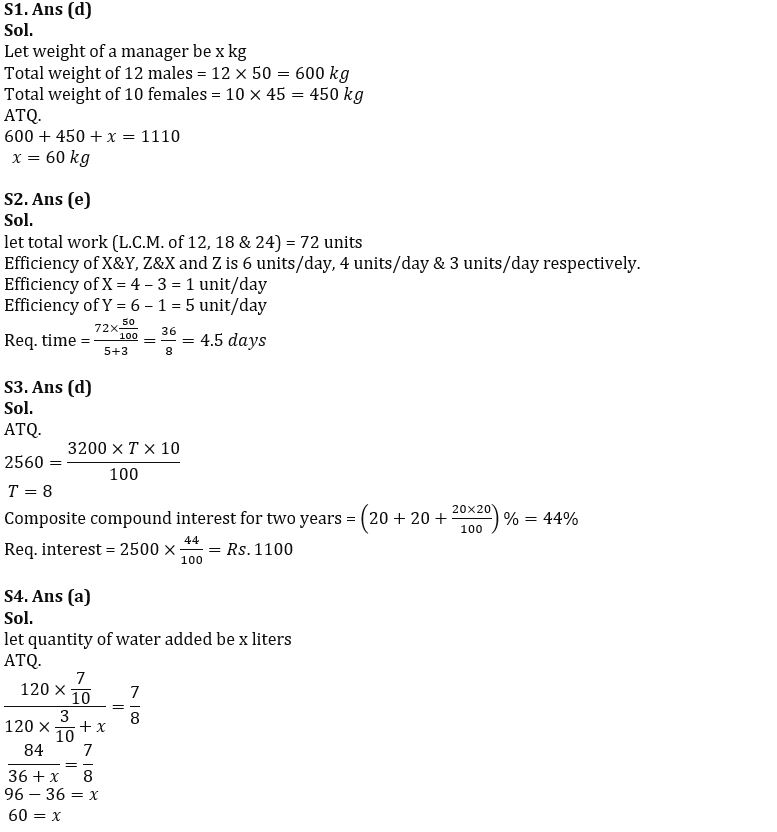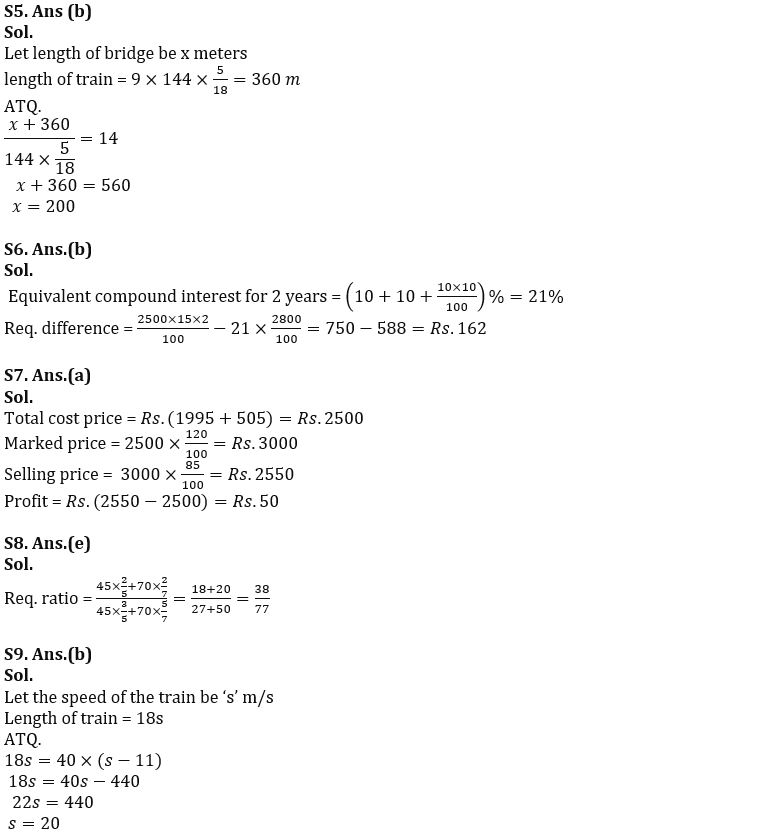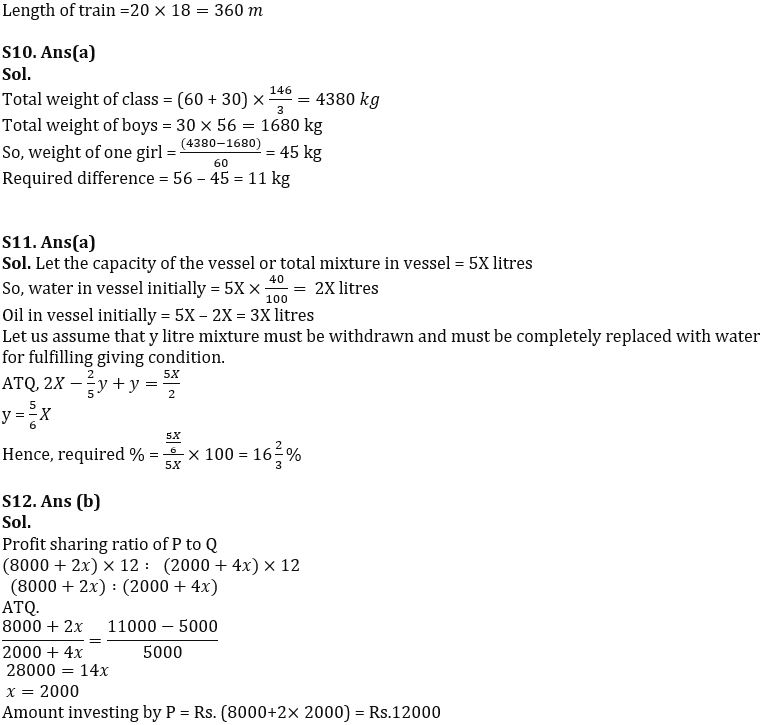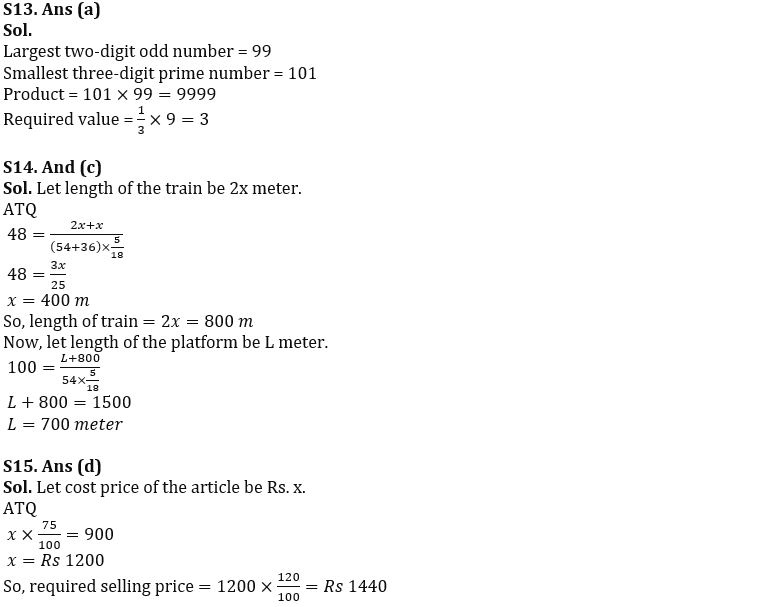Latest Banking jobs   »   Quantitative Aptitude Quiz

# Reasoning Quizzes Quiz For IRDA AM 2023- 15th April

Q1. In a HR department there are 12 males and 10 females. Average weight of each male and each female is 50 kg and 45 kg respectively. If the sum of weight of all the employees and a manager whose weight is ______ kg is 1110 kg, then what will come in the place of blank?
(a) 70
(b) 55
(c) 50
(d) 60
(e) 80

Q2. X & Y working together and Z & X working together can complete a work in 12 days and 18 days respectively. If Z alone can complete the same work in 24 days, then Y and Z together to finish 50% of work in ______ days, then what will come in the place of blank?
(a) 2
(b) 5
(c) 2.5
(d) 6
(e) 4.5

Q3. Rs.3200 when invested at S.I. of 10% p.a. for T years gives an interest of Rs.2560. If Rs.2500 is invested at C.I. compounding annually of 20% p.a. for (T-6) years, then C.I. is Rs, _________. What will come in the place of blank?
(a) 1500
(b) 1250
(c) 1420
(d) 1100
(e) 1080

Q4. A jar contains 120 liters mixture of wine and water in the ratio of 7:3 respectively. If ¬¬¬¬¬¬-______ liters of water are added in the mixture, then ratio of water to wine in the resultant mixture becomes 8:7 respectively. What will come in the place of blank?
(a) 60
(b) 68
(c) 70
(d) 54
(e) 50

Q5. A train crosses a bridge which length _______ meters in 14 seconds while it can cross a man standing on platform in 9 seconds. If the speed of the train is 144 km/hr, then what will come in the place of blank?
(a) 180
(b) 200
(c) 240
(d) 250
(e) 100

Q6. If Q invested Rs.2500 in scheme A which offers 15% simple interest for 2 years and Rs.2800 invested in scheme B which offers 10% C.I. for 2 years, then find the difference between interest earned from scheme A and scheme B.
(a) Rs.168
(b) Rs.162
(c) Rs.154
(d) Rs.148
(e) Rs.172

Q7. A shopkeeper buys an article for Rs.1995 and spent Rs.505 to transport that article. Shopkeeper marked 20% above the cost price. If he allows 15% discount on marked price, then find the profit earned by shopkeeper.
(a) Rs.50
(b) Rs.60
(c) Rs.20
(d) Rs.55
(e) Rs.75

Q8. 45 liters of mixture G and 70 liters of mixture Z contains milk and water in the ratio of 3:2 and 5:2 respectively. If mixture G and Z are mixed together, then what will be the ratio of quantity of water to quantity of milk in the resultant mixture.
(a) 38/79
(b) 32/77
(c) 39/77
(d) 31/77
(e) 38/77

Q9. If a train crosses a tree in 18 seconds and crosses a man running in same direction of train at the speed of 11 m/s in 40 seconds, then find the length of the train?
(a) 340 m
(b) 360 m
(c) 380 m
(d) 315 m
(e) 395 m

Q10. In a class there are 60 girls and 30 boys, and the total average weight of class is 48 2/3 kg. If the average weight of boys is 56 kg, then find the difference between the average weight of one boy and one girl?
(a) 11 kg
(b) 9 kg
(c) 15 kg
(d) 10 kg
(e) None of these

Q11. A vessel is fully-filled with mixture of water and oil. If in the mixture water is 40%, then how much quantity of mixture (in percent) must be drawn and completely replaced with water so that the resulting mixture contains half water and half oil?
(a) 16⅔%
(b) 33⅓%
(c) 46⅔%
(d) 73⅓%
(e) 86⅔%

Q12. P and Q started a business by investing Rs. (8000+2x) and Rs. (2000+4x) respectively. At the end of the year, the profit share of Q is Rs.5000 out of total profit of Rs. 11000, Find the total amount invested by P?
(a) Rs.14000
(b) Rs.12000
(c) Rs.18000
(d) Rs.12500
(e) Rs.11050

Q13. A is the largest two-digit odd number and B is smallest three-digit prime number. If X is the unit digit of product of A and B, then find the value of 100/3% of X?
(a) 3
(b) 5
(c) 9
(d) 12
(e) 1

Q14. A train travelling at 54 kmph crosses another train, having half of its length and travelling in opposite direction at 36 kmph in 48 seconds. It also passed a railway platform in 100 seconds. Find the length of the platform.
(a) 400 m
(b) 600 m
(c) 700 m
(d) 900 m
(e) 500 m

Q15. By selling an article at Rs 900, a shopkeeper gets a loss of 25%. Find, to make a profit of 20% he should sell the article at how much price?
Rs 1200
Rs 1320
Rs 1180
Rs 1440
Rs 1560

Solutions## FAQs

### How many sections are there in the IRDAI exam?

There are 4 sections in the IRDAI assistant manager online preliminary exam. They are Reasoning, English Language, General awareness, Quantitative aptitude.

#### Congratulations!Union Budget 2023-24: Free PDF